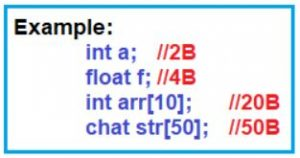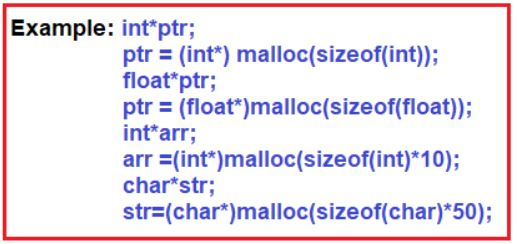# Dynamic Memory Management in C

## Dynamic Memory Management in C Language

In this article, I am going to discuss Dynamic Memory Management in C Language with Examples. Please read our previous articles, where we discussed Typedef in C. As part of this article, you will learn Memory Management in C and it’s typed with examples.

##### Memory Management in C

In the C Programming language, we are having two types of Memory Management

1. Static Memory Management
2. Dynamic Memory Management
##### Static Memory Management in C Language
1. When we are creating the memory at the time of compilation then it is called static Memory Management or Compile-time Memory Management. Static Memory Allocation is under control of Compiler.
2. When we are working with Static Memory Allocation, it is not possible to extend the memory at the time of execution, if it is not sufficient.
3. When we are working with static memory allocation, we need to go for pre-allocation of memory i.e. how many bytes of data required to be created is needed to be decided using coding only.##### Dynamic Memory Management in C Language
1. It is a procedure of allocating or de-allocating the memory at the run time i.e. dynamically. By using Dynamic Memory Allocation, we can utilize the memory more efficiently according to the requirement.
2. By using dynamic Memory allocation, whenever we want, which type we want, and how much we want, that time, type, and that much we can create dynamically.

Note: Dynamic Memory Allocation related all predefined functions are declared in <malloc.h>, <alloc.h>,<stdio.h>

#### Dynamic Memory Allocation Related Predefined Functions in C Language:

##### malloc() function in C:

By using this predefined function, we can create the memory dynamically at initial stage. malloc() function require 1 argument of type size_type i.e., datatype size. malloc() creates memory in bytes format and initial value is garbage.

Syntax: void *malloc(size_type);

Dynamic Memory Allocation related functions can be applied for any datatype that’s why functions return void* i.e. generic type. When we are working with Dynamic Memory Allocation related functions, we are required to perform Type Casting because functions return void*.##### Program for Dynamic Memory Allocation using malloc() Function in C Language
```#include <stdio.h>
#include <stdlib.h>

int main ()
{
int *ptr;
int n, i;
n = 5;
printf ("Enter number of elements: %d\n", n);
ptr = (int *) malloc (n * sizeof (int));

if (ptr == NULL)
{
printf ("Memory not allocated.\n");
exit (0);
}
else
{
printf ("Memory successfully allocated using malloc.\n");
for (i = 0; i < n; ++i)
{
ptr[i] = i + 1;
}

printf ("The elements of the array are: ");
for (i = 0; i < n; ++i)
{
printf ("%d, ", ptr[i]);
}
}
return 0;
}
```
###### Output:##### free() function in C Language:

By using this predefined function, we can deallocate dynamically allocated memory. When we are working with Dynamic /memory Allocation related memory, it stores in the heap area of the data segment and it is permanent memory if we are not deallocating. When we are working with Dynamic Memory Allocation related programs, at the end of the program recommended to deallocate memory by using the free() function. free() function requires one argument of type (void*) and returns void type.

Syntax: void free(void *ptr);

##### Program for Memory Deallocation using the free function in C Language
```#include<stdio.h>
#include<conio.h>
#include<malloc.h>
int main ()
{
int *arr;
int sum = 0, i, size;
float avg;
printf ("\n enter array size:");
scanf ("%d", &size);
arr = (int *) malloc (sizeof (int) * size);
printf ("\n Default Values : ");
for (i = 0; i < size; i++)
printf ("%d", arr[i]);
printf ("\n enter %d values : ", size);
for (i = 0; i < size; i++)
{
scanf ("%d", &arr[i]);
sum += arr[i];
}
avg = (float) sum / size;
printf ("\n sum of List : %d", sum);
printf ("\n Avg of List : 5.2f", avg);
free (arr);
arr = NULL;
return 0;
}
```
###### Output:##### Calloc Function in C Language:

By using this predefined function, we can create the memory dynamically at the initial stage. calloc() requires two argument of type (count, size_type). the count will provide a number of elements, size_type is datatype size. when we are working will calloc() function, it creates the memory in block format and the initial value is zero.

Syntax: void *calloc(count, size_type);##### Program for Dynamic Memory Allocation using calloc() Function in C Language
```#include <stdio.h>
#include <stdlib.h>
int main ()
{
int *ptr;
int n, i;
n = 5;
printf ("Enter number of elements: %d\n", n);
ptr = (int *) calloc (n, sizeof (int));

if (ptr == NULL)
{
printf ("Memory not allocated.\n");
exit (0);
}
else
{
printf ("Memory successfully allocated using calloc.\n");
for (i = 0; i < n; ++i)
{
ptr[i] = i + 1;
}

printf ("The elements of the array are: ");
for (i = 0; i < n; ++i)
{
printf ("%d, ", ptr[i]);
}
}
return 0;
}
```
###### Output:##### Realloc() Function in C Language:

By using this predefined function, we can create the memory dynamically at the middle stage of the program. Generally, this function is required to use when we are reallocating memory. realloc() requires two arguments of type void*, size_type. void* indicates previous block base address, size_type is datatype size. When we are working with realloc() function, it creates the memory in bytes format and the initial value is garbage.

Syntax: void *realloc(void*, size_type)##### Program for Dynamic Memory Allocation using realloc Function in C Language
```#include<stdio.h>
#include<conio.h>
#include<malloc.h>
int main ()
{
int *arr;
int s1, s2, i;

printf ("\n Enter array size1:");
scanf ("%d", &s1);
arr = (int *) calloc (s1, sizeof (int));
printf ("\n Enter %d values:", s1);
for (i = 0; i < s1; i++)
scanf ("%d", &arr[i]);

printf ("\n Enter array size2:");
scanf ("%d", &s2);
arr = (int *) realloc (arr, sizeof (int) * (s1 + s2));
printf ("\n Enter %d values", s2);
for (i = s1; i < s1 + s2; i++)
scanf ("%d", &arr[i]);

printf ("\n Array Data List :");
for (i = 0; i < s1 + s2; i++)
printf ("%d ", arr[i]);

printf ("%d", arr[i]);
free (arr);
arr = NULL;
return 0;
}
```
###### Output: